## Putting It Together: Sequences and Series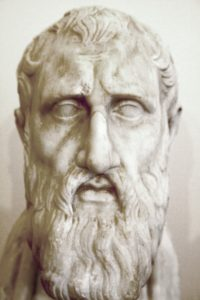Marble bust of Zeno of Citium.

The Greek philosopher Zeno of Elea (c. 490-450 BC) described certain everyday scenarios, such as walking down a path or shooting an arrow, in ways that made them seem impossible to accomplish.  These stories are called Zeno’s Paradoxes.  The word paradox itself means self-contradictory statement, and Zeno’s original intent in telling these paradoxes was to argue that motion is somehow an illusion.  However we can use what we have learned in this module to show that there is no self-contradiction at all.

Suppose you are at the beginning of a mile long path.  In order to walk the whole mile, certainly you will have to make it to the halfway point first.  After traversing that first half-mile, in order to complete the rest of the journey you will have to make it halfway through the next half-mile —  in other words, you will have to walk an additional quarter-mile.  Now that you have walked three quarters of a mile total, you’ll need to make it to the next halfway point, which is half of a quarter mile away, another 1/8th of a mile.  The journey continues as you cover an additional 1/16th, then 1/32nd, and then 1/64th of a mile, and so on.  Every time you get to the next halfway point, there will be a new halfway point looming ahead.

In other words, there are infinitely many halfway points to get past, and as Zeno argued, since no one can accomplish an infinite number of tasks, there is simply no way to walk the entire mile!  In fact, the same reasoning applies to any distance, no matter how short.  Motion itself seems to be impossible because the object in motion would have to pass an infinite number of halfway points before reaching its destination.

Now obviously people can and do walk various distances all the time.  Where does Zeno’s argument go wrong?  It was in his misunderstanding of an infinite series.  Let’s see what the journey looks like at each stage, adding up all of the distances in turn.  We’ll keep track of our progress on a number line.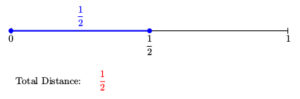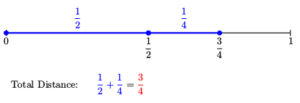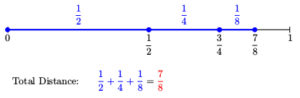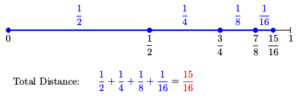As you can see, at every stage the total distance only grows by smaller and smaller amounts.  In fact, we are adding the terms of a geometric series whose common ratio is equal to $r={\large\frac{1}{2}}$.

But the process doesn’t really stop, right?  What would be the sum of infinitely many terms of the series?

Let $S = \dfrac{1}{2} + \dfrac{1}{4} + \dfrac{1}{8} + \dfrac{1}{16} + \dfrac{1}{32} + \cdots$, which represents the sum of all stages of the journey.

Here, we have initial term $a_1={\large\frac{1}{2}}$, and because $-1 < r < 1$, we can use the formula for the sum of an infinite geometric series.

$S ={\Large\frac{a_1}{1 - r}}={\Large\frac{\frac{1}{2}}{1 - \frac{1}{2}}}={\Large\frac{\left(\frac{1}{2}\right)}{\left(\frac{1}{2}\right)}}= 1.$

Notice that the formula correctly sums the infinitely many distances that make up the full mile.  What Zeno did not realize is that an infinite series could have a finite sum!